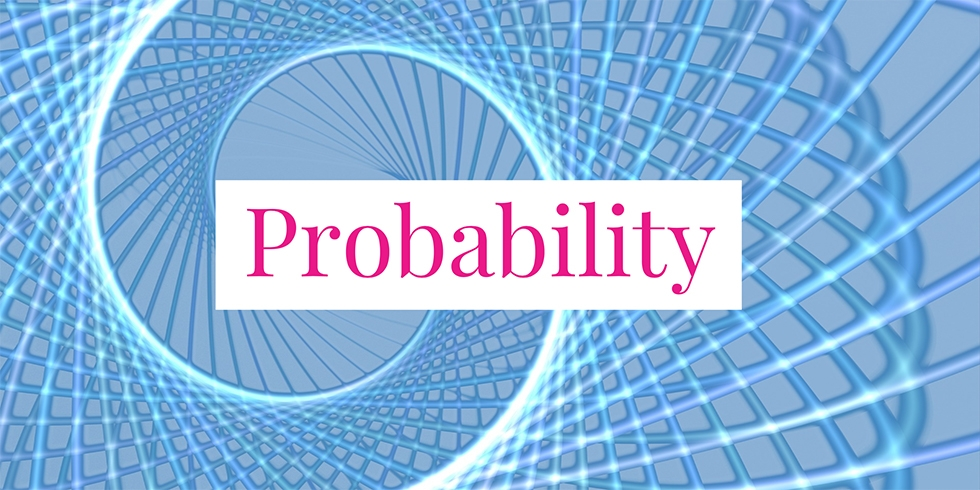# Complement of Independent Events are Independent## Problem 734

Let $E$ and $F$ be independent events. Let $F^c$ be the complement of $F$.

Prove that $E$ and $F^c$ are independent as well.Add to solve later

## Solution.

Note that $E\cap F$ and $E \cap F^c$ are disjoint and $E = (E \cap F) \cup (E \cap F^c)$. It follows that
$P(E) = P(E \cap F) + P(E \cap F^c).$ As $E$ and $F$ are independent, we know that
$P(E \cap F) = P(E)\cdot P(F)$ Combining these two equalities, we get
\begin{align*}
P(E \cap F^c) &= P(E) – P(E \cap F)\\
&= P(E) – P(E) \cdot P(F)\\
&= P(E)(1-P(F)).
\end{align*}

Since $P(F^c) = 1 – P(F)$, we obtain the equality
$P(E \cap F^c) = P(E)\cdot P(F^c),$ which implies that $E$ and $F^c$ are independent.

### Remark

We just proved that when $E$ and $F$ are independent events, then $E$ and the complement $F^c$ are independent.
Now, we apply this statement to the independent events $E$ and $F^c$. Then we see that the complements $E^c$ and $F^c$ are independent.

In conclusion, if two events are independent, then their complements are also independent.Add to solve later

### More from my site

#### You may also like...

This site uses Akismet to reduce spam. Learn how your comment data is processed.

###### More in Probability##### Independent and Dependent Events of Three Coins Tossing

Suppose that three fair coins are tossed. Let $H_1$ be the event that the first coin lands heads and let...

Close